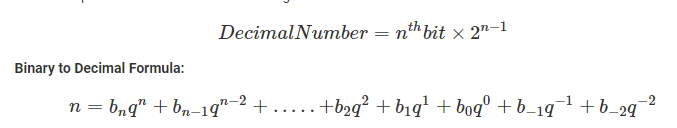# Binary to Decimal Conversion

Binary to decimal conversion explains the conversion of a binary number (base-2) to an equivalent decimal number (base-10). In Mathematics, a number system is used for expressing numbers. It is a way to represent numbers. The four different types of number system are:

• Binary Number System (Base-2)
• Octal Number System (Base-8)
• Decimal Number System (Base-10)

Number system plays an essential role in computer architecture. Not only computer architecture but mostly all electronic gadgets also uses the number system. So it is essential to learn the conversion from one number system to the other number system. Before we discuss about binary to decimal conversion, let us first understand both the number systems.

## What is a Binary Number System?

A binary number system is defined as a number that is used in binary systems. It is also called base 2 numeral system. It represents the numeric values with two distinct symbols, basically 1 (one) and 0 (zero).

## What is a Decimal Number System?

The decimal number system is also known as the base 10 numeral system. It uses ten digits from 0 to 9. In the decimal number system, the positions continuous to the left of the decimal point represent units, tens, hundreds, thousands and so on. Thus, the base of the decimal number system is 10.

## What is Binary to Decimal Conversion?

Binary to decimal conversion is done to convert a number in a binary number system (base-2) to a number in a decimal number system (base-10). It is very necessary to understand the binary to decimal conversion for computer programming applications. The binary number system is represented by only two digits i.e., 0 and 1 whereas the decimal number system include all the 10 digits from 0 to 9. Now, we have to learn how to convert a binary number to the corresponding decimal number in the next section.

## How to Convert Binary to Decimal Numbers?

To convert the binary number to a decimal number, we use the multiplication method. In this conversion process, if a number with base n has to be converted into a number with base 10, then each digit of the given number is multiplied from the Most Significant Bit (MSB) to the Least Significant Bit (LSB) with reducing the power of the base.

### Binary to Decimal Conversion Steps

• First, write the given binary number and count the powers of 2 from right to left (powers starting from 0)
• Now, write each binary digit (right to left) with the corresponding powers of 2 from (right to left), such that first binary digit (MSB) will be multiplied with the greatest power of 2.
• Add all the products in the above step
• The final answer will be the required decimal number

Let us understand this conversion with the help of an example.

Example of Binary to Decimal Conversion:

Convert the binary number (1101)2 into a decimal number.

Solution:

Given binary number = (1101)2

Now, multiplying each digit from MSB to LSB with reducing the power of the base number 2.

1 × 23 + 1 × 22 + 0 × 21 + 1 × 20

= 8 + 4 + 0 + 1

= 13

Thus, the equivalent decimal number for the given binary number (1101)2 is (13)10

Also, Check: Binary to Decimal Calculator

## Binary to Decimal Formula

The formula for the conversion of the binary number to the decimal number is given by:Where,

N is decimal equivalent,

b is the digit,

q is the base value

## Binary to Decimal Table

The conversion chart for the binary to the decimal number is given below.

 Binary Decimal 0 0 1 1 10 2 11 3 100 4 101 5 110 6 111 7 1000 8 1001 9 1010 10 1011 11 1100 12

## Solved Examples

Q.1: Convert the binary number 1001 to a decimal number.

Solution: Given, binary number = 10012

Hence, using the binary to decimal conversion formula, we have:

10012 = (1 × 2³) + (0 × 2²) + (0 × 2¹) + (1 × 2⁰)

= 8 + 0 + 0 + 1

= (9)₁₀

Q.2: Convert 11010012 into an equivalent decimal number.

Solution: Using binary to decimal conversion method, we get;

(1101001)₂ = (1 × 2⁶) + (1 × 2⁵) + (0 × 2⁴) + (1 × 2³) + (0 × 2²) + (0 × 2¹) + (1 × 2⁰)

= 64 + 32 + 0 + 8 + 0 + 0 + 1

= (105)₁₀

Q.3: Convert (11110111)2 into base-10 number system.

Solution: Using binary to decimal conversion method, we get;

(11110111)₂ = (1 × 2⁷) + (1 × 2⁶) + (1 × 2⁵) + (1 × 2⁴) + (0 × 2³) + (1 × 2²) + (1 × 2¹) + (1 × 2⁰)

= 128 + 64 + 32 + 16 + 0 + 4 + 2 + 1

= (247)₁₀

## Practice Questions

1. Convert binary number 10111 into an equivalent decimal number.
2. Convert 1112 in decimal number.
3. What is 1010102 in decimal number?

To learn more on the number systems, register with BYJU’S – The Learning App and download the app from the Google Play store to watch all the subject related videos to learn with ease.

Quiz on Binary to Decimal